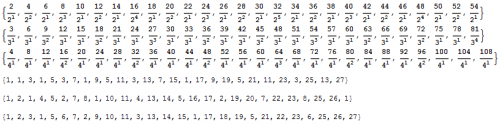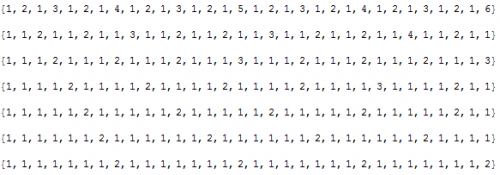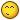## Recommended Posts

In my sixth challenge, which is based upon the Collatz conjecture, I found a way to determine the odd numbers that reduce to one given a count of the odd numbers within their Hailstone sequences. The reason why I focused my efforts on odd numbers is because all even numbers will reduce to an odd number by dividing it by two until an odd number is reached. As I was analyzing the sequence of exponents generated by recursively dividing even numbers by two, I noticed an interesting pattern and derived a formula to predict these exponents. Not only did I discover how to predict powers of two, but also for any other factor as well. The following image shows the exponents for factors of two, three, and four:As you can see, the first sequence is based on factors of two for the function $f(x)=2\,x$, the second sequence is based on factors of three for the function $f(x)=3\,x$, etc... Of course, the inputs and outputs are restricted to the set of integers. The challenge is to find a formula that predicts these exponent given the $x$ location and the factor. In other words, find the equation that predicts the values of $n$ given a factor $a$ and the variable $x$ for

$\frac{a\, x}{a^n}$

Reputation awaits the one who can complete the challenge first.

Edited by Daedalus

##### Share on other sites

Instead of looking at all of the numbers as if they are all part of the same group(though they are), look at them individually.This will help in the development of an equation. Ask questions if needed.

And notice how the order seems to correlate with each other.

n+4: 1->

6/2 = 3, 10/2 = 5, 14/2 = 7, 18/2 = 9...

n+8: 2->

12/4 = 3, 20/4 = 5, 28/4 = 7, 36/4 = 9...

n+16: 3->

24/8 = 3, 40/8 = 5...

n+32: 4->

48/16 = 3...

Edited by Unity+

##### Share on other sites

Don't forget that you have to provide a single formula that works for any factor and value of $x$. Not just powers of two.

Just so there is no confusion as to what numbers your formula should generate, I am posting this image showing the exponents for factors of 2 through 8.##### Share on other sites

Any takers? This one isn't too hard. Just a little tricky. It also has some interesting consequences.

Edited by Daedalus

##### Share on other sites

It has been over a week since I posted the challenge, and it's time for a hint. Due to the cyclic nature of the problem, the solution involves using the modulo operation. However, I prefer to use the floor function to get the remainder:

$r=a-n\,q=a-n\left\lfloor\frac{a}{n}\right\rfloor$

##### Share on other sites

I realize now that after having to write the solution for this challenge, that it was probably harder to solve than most of my challenges. Nevertheless, I am posting the solution because no one solved it, or shown interest in trying to solve it.When I analyzed the sequence of exponents for the factors 2 through 8, I noticed that the ones repeat themselves in a regular pattern. This led me to look for a pattern in the twos, threes, and so forth. As expected, the exponents cover the entire set of natural number and repeat in regular patterns.

{1, 2, 1, 3, 1, 2, 1, 4, 1, 2, 1, 3, 1, 2, 1, 5, 1, 2, 1, 3, 1, 2, 1, 4, 1, 2, 1, 3, 1, 2, 1, 6, ...}
{1, 1, 2, 1, 1, 2, 1, 1, 3, 1, 1, 2, 1, 1, 2, 1, 1, 3, 1, 1, 2, 1, 1, 2, 1, 1, 4, 1, 1, 2, 1, 1, ...}
{1, 1, 1, 2, 1, 1, 1, 2, 1, 1, 1, 2, 1, 1, 1, 3, 1, 1, 1, 2, 1, 1, 1, 2, 1, 1, 1, 2, 1, 1, 1, 3, ...}
{1, 1, 1, 1, 2, 1, 1, 1, 1, 2, 1, 1, 1, 1, 2, 1, 1, 1, 1, 2, 1, 1, 1, 1, 3, 1, 1, 1, 1, 2, 1, 1, ...}
{1, 1, 1, 1, 1, 2, 1, 1, 1, 1, 1, 2, 1, 1, 1, 1, 1, 2, 1, 1, 1, 1, 1, 2, 1, 1, 1, 1, 1, 2, 1, 1, ...}
{1, 1, 1, 1, 1, 1, 2, 1, 1, 1, 1, 1, 1, 2, 1, 1, 1, 1, 1, 1, 2, 1, 1, 1, 1, 1, 1, 2, 1, 1, 1, 1, ...}
{1, 1, 1, 1, 1, 1, 1, 2, 1, 1, 1, 1, 1, 1, 1, 2, 1, 1, 1, 1, 1, 1, 1, 2, 1, 1, 1, 1, 1, 1, 1, 2, ...}

Let's look at the sequence of exponents for factors of two.

{1, 2, 1, 3, 1, 2, 1, 4, 1, 2, 1, 3, 1, 2, 1, 5, 1, 2, 1, 3, 1, 2, 1, 4, 1, 2, 1, 3, 1, 2, 1, 6, ...}

We can easily see that the ones begin at the first position and repeat every other number. The twos begin at the second position and repeat every four numbers, and the threes begin at the fourth position and repeat every eight numbers. If we chart this out we'll get:

{1, 2, 1, 3, 1, 2, 1, 4, 1, 2, 1, 3, 1, 2, 1, 5, 1, 2, 1, 3, 1, 2, 1, 4, 1, 2, 1, 3, 1, 2, 1, 6, ...}
{1, 2, 1, 3, 1, 2, 1, 4, 1, 2, 1, 3, 1, 2, 1, 5, 1, 2, 1, 3, 1, 2, 1, 4, 1, 2, 1, 3, 1, 2, 1, 6, ...}
{1, 2, 1, 3, 1, 2, 1, 4, 1, 2, 1, 3, 1, 2, 1, 5, 1, 2, 1, 3, 1, 2, 1, 4, 1, 2, 1, 3, 1, 2, 1, 6, ...}
{1, 2, 1, 3, 1, 2, 1, 4, 1, 2, 1, 3, 1, 2, 1, 5, 1, 2, 1, 3, 1, 2, 1, 4, 1, 2, 1, 3, 1, 2, 1, 6, ...}

$\begin{matrix}\text{exp}&\text{start}&\text{spacing}\\1&1&2\\2&2&4\\3&4&8\\4&8&16\\...&...&...\end{matrix}$

If you look at the sequence of exponents for factors of three, you will notice that the ones are doubled up in the sequence:

{1, 1, 2, 1, 1, 2, 1, 1, 3, 1, 1, 2, 1, 1, 2, 1, 1, 3, 1, 1, 2, 1, 1, 2, 1, 1, 4, 1, 1, 2, 1, 1, ...}

This means that there are two equations that predict the positions of the ones, twos, threes, etc... And once again, if we chart this out, we get:

{1, 1, 2, 1, 1, 2, 1, 1, 3, 1, 1, 2, 1, 1, 2, 1, 1, 3, 1, 1, 2, 1, 1, 2, 1, 1, 4, 1, 1, 2, 1, 1, ...}
{1, 1, 2, 1, 1, 2, 1, 1, 3, 1, 1, 2, 1, 1, 2, 1, 1, 3, 1, 1, 2, 1, 1, 2, 1, 1, 4, 1, 1, 2, 1, 1, ...}

{1, 1, 2, 1, 1, 2, 1, 1, 3, 1, 1, 2, 1, 1, 2, 1, 1, 3, 1, 1, 2, 1, 1, 2, 1, 1, 4, 1, 1, 2, 1, 1, ...}
{1, 1, 2, 1, 1, 2, 1, 1, 3, 1, 1, 2, 1, 1, 2, 1, 1, 3, 1, 1, 2, 1, 1, 2, 1, 1, 4, 1, 1, 2, 1, 1, ...}

{1, 1, 2, 1, 1, 2, 1, 1, 3, 1, 1, 2, 1, 1, 2, 1, 1, 3, 1, 1, 2, 1, 1, 2, 1, 1, 4, 1, 1, 2, 1, 1, ...}
{1, 1, 2, 1, 1, 2, 1, 1, 3, 1, 1, 2, 1, 1, 2, 1, 1, 3, 1, 1, 2, 1, 1, 2, 1, 1, 4, 1, 1, 2, 1, 1, ...}

$\begin{matrix}\text{exp}&\text{start}&\text{spacing}\\1&1&3\\1&2&3\\2&3&9\\2&6&9\\3&9&27\\3&18&27\\...&...&...\end{matrix}$

We can see that exponential equations govern the pattern as well as the start indices and spacings. Furthermore, if we had an equation that would produce the red highlighted numbers in their exact position, but be zero everywhere else, then we could sum all the sequences together to have a generating function.

{1, 0, 1, 0, 1, 0, 1, 0, 1, 0, 1, 0, 1, 0, 1, 0, 1, 0, 1, 0, 1, 0, 1, 0, 1, 0, 1, 0, 1, 0, 1, ...} +
{0, 2, 0, 0, 0, 2, 0, 0, 0, 2, 0, 0, 0, 2, 0, 0, 0, 2, 0, 0, 0, 2, 0, 0, 0, 2, 0, 0, 0, 2, 0, ...} +
{0, 0, 0, 3, 0, 0, 0, 0, 0, 0, 0, 3, 0, 0, 0, 0, 0, 0, 0, 3, 0, 0, 0, 0, 0, 0, 0, 3, 0, 0, 0, ...} +
{0, 0, 0, 0, 0, 0, 0, 4, 0, 0, 0, 0, 0, 0, 0, 0, 0, 0, 0, 0, 0, 0, 0, 4, 0, 0, 0, 0, 0, 0, 0, ...} =

{1, 2, 1, 3, 1, 2, 1, 4, 1, 2, 1, 3, 1, 2, 1, 5, 1, 2, 1, 3, 1, 2, 1, 4, 1, 2, 1, 3, 1, 2, 1, ...}

This is where the modulo operation comes into play. If we use Mathematica, we can see that Mod[x,2] generates the sequence of ones as found in the exponents for the factors of two.

{1, 0, 1, 0, 1, 0, 1, 0, 1, 0, 1, 0, 1, 0, 1, 0, 1, 0, 1, 0, ...}

However, the sequence generated by Mod[x,3] has a bunch of twos in it, and we need a sequence of ones and zeros.

{1, 2, 0, 1, 2, 0, 1, 2, 0, 1, 2, 0, 1, 2, 0, 1, 2, 0, 1, 2, ...}

Here's the trick. We know that Mod[1,3] = 1 and that Mod[3x, 3]=0. So if we take the factor 3 and raise it to the power of Mod[x,3], $3^{\text{Mod[}x\text{,3]}}$, we'll get a one when Mod[x,3]=0, and a multiple of 3 everywhere else:

{3, 9, 1, 3, 9, 1, 3, 9, 1, 3, 9, 1, 3, 9, 1, 3, 9, 1, 3, 9, ...}

If we apply the modulo operation again, we get $\text{Mod[ }3^{\text{Mod[}x\text{, 3]}}\text{, 3 ]}$ :

{0, 0, 1, 0, 0, 1, 0, 0, 1, 0, 0, 1, 0, 0, 1, 0, 0, 1, 0, 0, ...}

This allows us to generate a one every three numbers and a zero everywhere else. This works for any factor, and by using the floor function instead of the modulo operator, we arrive at a function that generates any spacing of ones that we need with zeroes everywhere else:

$\text{FactorMap}\left(x, n\right)=n\left(\frac{n^{x-1}}{n^{n\left\lfloor\frac{x}{n}\right\rfloor}}-\left\lfloor\frac{n^{x-1}}{n^{n\left\lfloor\frac{x}{n}\right\rfloor}}\right\rfloor\right)$

If you prefer, you can still use the following in Mathematica:

$\text{FactorMap}\left(x, n\right)=\text{Mod[ }n^{\text{Mod[}x\text{, n]}}\text{, n ]}$

The reason I call this function the FactorMap is because it produces a 1 whenever a number contains the factor or a zero otherwise. Furthermore, if we sum the sequences produced by the function, we'll get a count of unique factors for each number.

numbers

1 2 3 4 5 6 7 8 9 ...

2 {0, 1, 0, 1, 0, 1, 0, 1, 0, 1, 0, 1, 0, 1, 0, 1, 0, 1, 0, 1}

3 {0, 0, 1, 0, 0, 1, 0, 0, 1, 0, 0, 1, 0, 0, 1, 0, 0, 1, 0, 0}

Factors: 4 {0, 0, 0, 1, 0, 0, 0, 1, 0, 0, 0, 1, 0, 0, 0, 1, 0, 0, 0, 1}

5 {0, 0, 0, 0, 1, 0, 0, 0, 0, 1, 0, 0, 0, 0, 1, 0, 0, 0, 0, 1}

6 {0, 0, 0, 0, 0, 1, 0, 0, 0, 0, 0, 1, 0, 0, 0, 0, 0, 1, 0, 0}

Now that we have this function, we can choose the correct spacing, multiply it by the value of our exponents, and set the correct start indices. By using the charts we made earlier, we can derive such a function ($i$ and $j$ are indices used to offset to the correct start position and choose the proper spacing):

$F(x,n,i,j)=j\,\text{FactorMap}\left(x-i\,n^{j-1}, n^{j}\right)$

The only thing left is to determine how many times we need to sum $F$ in order to create the generating function. The key is knowing where the ones, twos, threes, etc... start. Look at the sequence of exponents for factors of two:

{1, 2, 1, 3, 1, 2, 1, 4, 1, 2, 1, 3, 1, 2, 1, 5, 1, 2, 1, 3, 1, 2, 1, 4, 1, 2, 1, 3, 1, 2, 1, 6, ...}

Since every exponent is represented by its own equation, we would have to add a sum whenever a one, two, three, etc..., occurred. The following formula accomplishes this feat for any factor, $n$:

$\left\lfloor\text{log}_{\,n}\,x\right\rfloor+1$

Regarding the sequence of exponents for factors of two, we can see this formula in action:

{1, 2, 1, 3, 1, 2, 1, 4, 1, 2, 1, 3, 1, 2, 1, 5, 1, 2, 1, 3, 1, 2, 1, 4, 1, 2, 1, 3, 1, 2, 1, 6, ...}

{1, 2, 2, 3, 3, 3, 3, 4, 4, 4, 4, 4, 4, 4, 4, 5, 5, 5, 5, 5, 5, 5, 5, 5, 5, 5, 5, 5, 5, 5, 5, 6, ...}

Now we can put all of the pieces of the puzzle together, and arrive at the solution for the challenge:

$\text{Factors}(x, n)=\sum_{i=1}^{n-1}\left(\sum_{j=1}^{\left\lfloor\text{log}_{\,n}\,x\right\rfloor+1}F(x,n,i,j)\right)$

As a bonus, if we subtract one from our Factors function, then we get an algorithm that can tell us how many times a factor occurs.

$\text{Factors}(x, n)=\sum_{i=1}^{n-1}\left(\sum_{j=1}^{\left\lfloor\text{log}_{\,n}\,x\right\rfloor+1}F(x,n,i,j)\right)-1$

numbers

1 2 3 4 5 6 7 8 9 10 ...

2 {0, 1, 0, 2, 0, 1, 0, 3, 0, 1, 0, 2, 0, 1, 0, 4, 0, 1, 0, 2, 0, 1, 0, 3, 0, 1, 0, 2, 0, 1, 0, 5}

3 {0, 0, 1, 0, 0, 1, 0, 0, 2, 0, 0, 1, 0, 0, 1, 0, 0, 2, 0, 0, 1, 0, 0, 1, 0, 0, 3, 0, 0, 1, 0, 0}

4 {0, 0, 0, 1, 0, 0, 0, 1, 0, 0, 0, 1, 0, 0, 0, 2, 0, 0, 0, 1, 0, 0, 0, 1, 0, 0, 0, 1, 0, 0, 0, 2}

Factors: 5 {0, 0, 0, 0, 1, 0, 0, 0, 0, 1, 0, 0, 0, 0, 1, 0, 0, 0, 0, 1, 0, 0, 0, 0, 2, 0, 0, 0, 0, 1, 0, 0}

6 {0, 0, 0, 0, 0, 1, 0, 0, 0, 0, 0, 1, 0, 0, 0, 0, 0, 1, 0, 0, 0, 0, 0, 1, 0, 0, 0, 0, 0, 1, 0, 0}

7 {0, 0, 0, 0, 0, 0, 1, 0, 0, 0, 0, 0, 0, 1, 0, 0, 0, 0, 0, 0, 1, 0, 0, 0, 0, 0, 0, 1, 0, 0, 0, 0}

8 {0, 0, 0, 0, 0, 0, 0, 1, 0, 0, 0, 0, 0, 0, 0, 1, 0, 0, 0, 0, 0, 0, 0, 1, 0, 0, 0, 0, 0, 0, 0, 1}

I've attached the Mathematica 7 file for those who wish to download it.FactorMap.zip

Edited by Daedalus

## Create an account

Register a new account